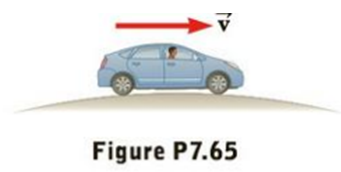Chapter 7, Problem 65AP

Chapter
Section
Textbook Problem

Suppose a 1 800-kg car passes over a bump in a roadway that follows the arc of a circle of radius 20.4 m, as in Figure P7.65. (a) What force does the road exert on the car as the car passes the highest point of the bump if the car travels at 8.94 m/s? (b) What is the maximum speed the car can have without losing contact with the road as it passes this highest point?Figure P7.65

a)

To determine
The force exerted by the road on the car as the car passes the highest point of the bump.

Explanation

Given info: The mass of the car is 1800kg . The radius of the circular arc is 20.4m . The speed of the car at the highest point is 8.94ms-1 .

Explanation:

The resultant of the normal force and the weight of the car are providing the required centripetal acceleration. The weight is directed vertically downward and the normal force is vertically upward. The resultant force must direct downward to provide the required centripetal acceleration.

mgN=mv2r

• r is the radius of the circular arc
• N is the normal force on the car by the road
• m is the mass of the car
• v is the velocity of the car

The normal force on the car will be,

N=m(gv2r)

Substitute 20.4m for r , 8.94ms-1 for v , 9

b)

To determine
The maximum speed the car can have without losing the contact with the road as it passes through the highest point on the road.

Still sussing out bartleby?

Check out a sample textbook solution.

See a sample solution

The Solution to Your Study Problems

Bartleby provides explanations to thousands of textbook problems written by our experts, many with advanced degrees!

Get Started